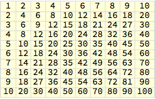Try it now »
(no sign-in required)# Multiplication Tables

Instantly make a multiplication table of any size.

Run the code to create the data for a 10×10 multiplication table. Try other arithmetic operations, like addition (+) or division (/):

Table makes lists of things.

Heres a list of 10 even numbers:

Table[a*2, {a, 10}]

If you make a table with two indices (a and b), you get a list of lists. This is the data for a multiplication table:

Table[a*b, {a, 10}, {b, 10}]

Table[a*b, {a, 10}, {b, 10}]

Lay out the numbers in a grid. Try making different sizes of grids:

Grid formats a list of lists as a neat grid:

Grid[{{1, 2, 3}, {4, 5, 6}}]

Grid is often used together with Table because Table makes lists of lists:

Grid[Table[a*b, {a, 10}, {b, 10}]]

Use FrameAll to put lines in the grid:

Grid[Table[a*b, {a, 10}, {b, 10}], Frame -> All]

Grid[Table[a*b, {a, 10}, {b, 10}], Frame -> All]

Add color. Try other colorsfor example LightGreen, LightOrange or LightGray:

Nearly every aspect of the appearance of grids can be customized. You can make the background light yellow:

Grid[Table[a*b, {a, 10}, {b, 10}], Frame -> All, Background -> LightYellow]

Lighten the grid lines with FrameStyleLightGray:

Grid[Table[a*b, {a, 10}, {b, 10}], Frame -> All, Background -> LightYellow, FrameStyle -> LightGray]

Grid[Table[a*b, {a, 10}, {b, 10}], Frame -> All, Background -> LightYellow, FrameStyle -> LightGray]

Use 8-point type. Try font sizes other than 8for example, 5:

You can customize the style of the items in the grid. This sets the size of the font to 8 points (a point is a printers measure equal to 1/72 inch):

Grid[Table[Style[a*b, 8], {a, 10}, {b, 10}], Frame -> All, Background -> LightYellow, FrameStyle -> LightGray]

You might want to make the font smaller if your grid is very large:

Grid[Table[Style[a*b, 8], {a, 25}, {b, 25}], Frame -> All, Background -> LightYellow, FrameStyle -> LightGray]

Grid[Table[Style[a*b, 8], {a, 30}, {b, 30}], Frame -> All, Background -> LightYellow, FrameStyle -> LightGray]

Share Itmake a multiplication table website:

Deploy a form that asks for a number and makes a multiplication table from 1 to that number:

CloudDeploy[FormFunction[{"n" -> "Integer"}, (Grid[Table[Style[a*b, 12], {a, #n}, {b, #n}], Frame -> All, Background -> LightYellow, FrameStyle -> LightGray]) &, "PNG"], "Permissions" -> "Public" ]

Click the link in the output to visit the site.